## Boost Converter – 3.3V@ 0.4A

Its time to show you my 3.3V output boost Converter design. In one of my earlier post I showed you step by step on how to design your own boost converter and if you haven’t read that yet then click here.

#### Lets get started:

##### Intro.

First of all, why do we even need this converter? Well every sensor, microcontroller, arduino, ESP8266, and various other digital components need a constant voltage.  A constant voltage is necessary to maintain proper operation of these components.

Here we will see the advantage of this boost converter.

##### Specifications.

Below are the operating specs for this converter$\Huge \bold V_\text{IN} = 1.8V - 2.4V$$\Huge \bold V_\text{OUT} = 3.3V$$\Huge \bold I_\text{OUT} = 0.4A$

• Note: Different Vin voltages gives you different max power output
•$\Huge \bold V_\text{IN} = 1.8V @ I_\text{OUT}: 0.2A$
•$\Huge \bold V_\text{IN}: 2.0V @ Iout: 0.3A$
•$\Huge \bold V_\text{IN}: 2.4V @ Iout: 0.4A$$\Huge \bold V_\text{P-P} = 80mV$

##### Bill of Materials.

Here is a screenshot of the bill of materials.  I added the suppliers on the spreadsheet because I’ve found that some sites have better pricing than others.

Using octopart.com, you can actually find the best value for the component you’re looking for.  I highly suggest you go look at the site.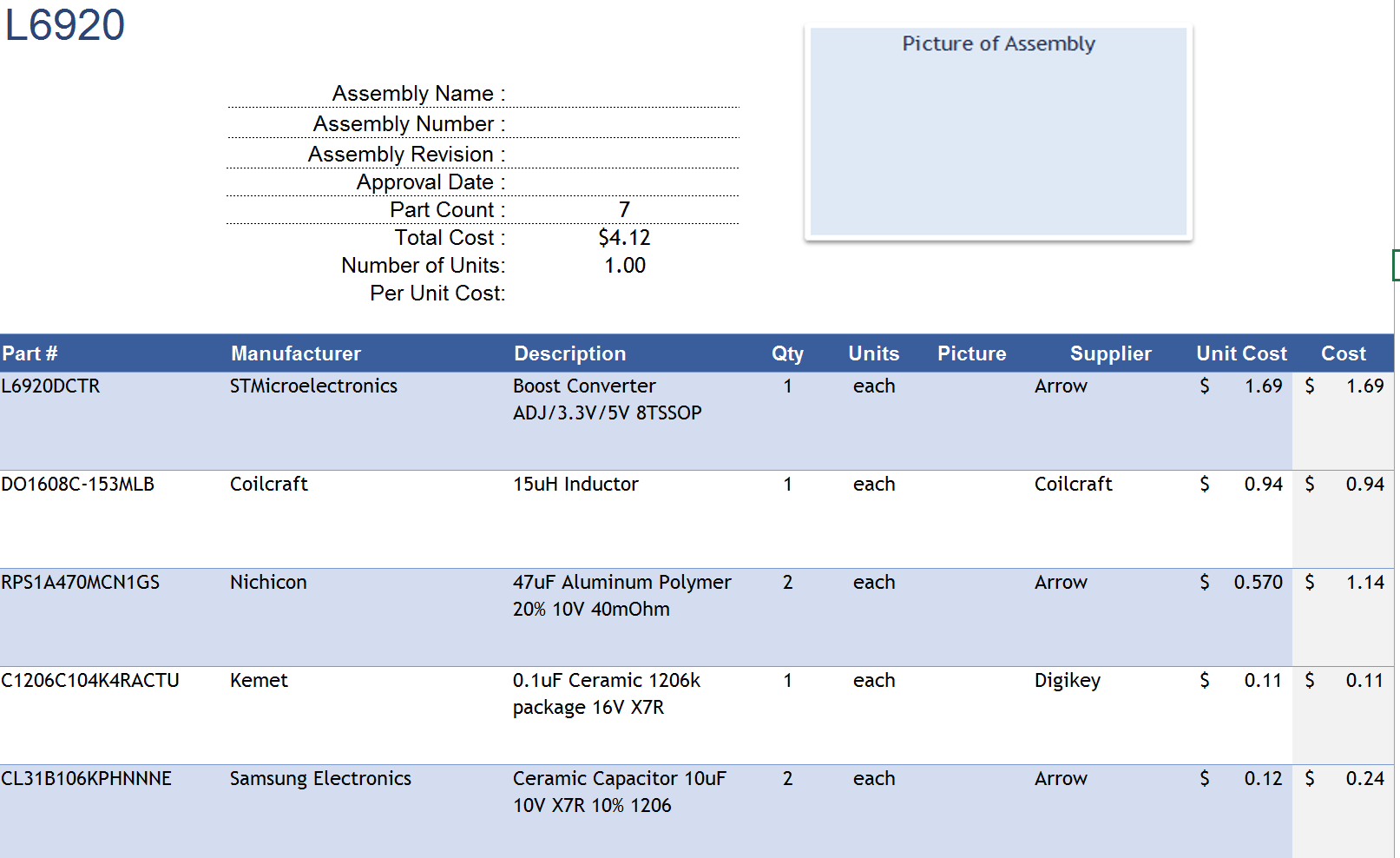##### Schematic.

Attached here is the schematic for this project.  All the original files are available for download at the bottom of the page.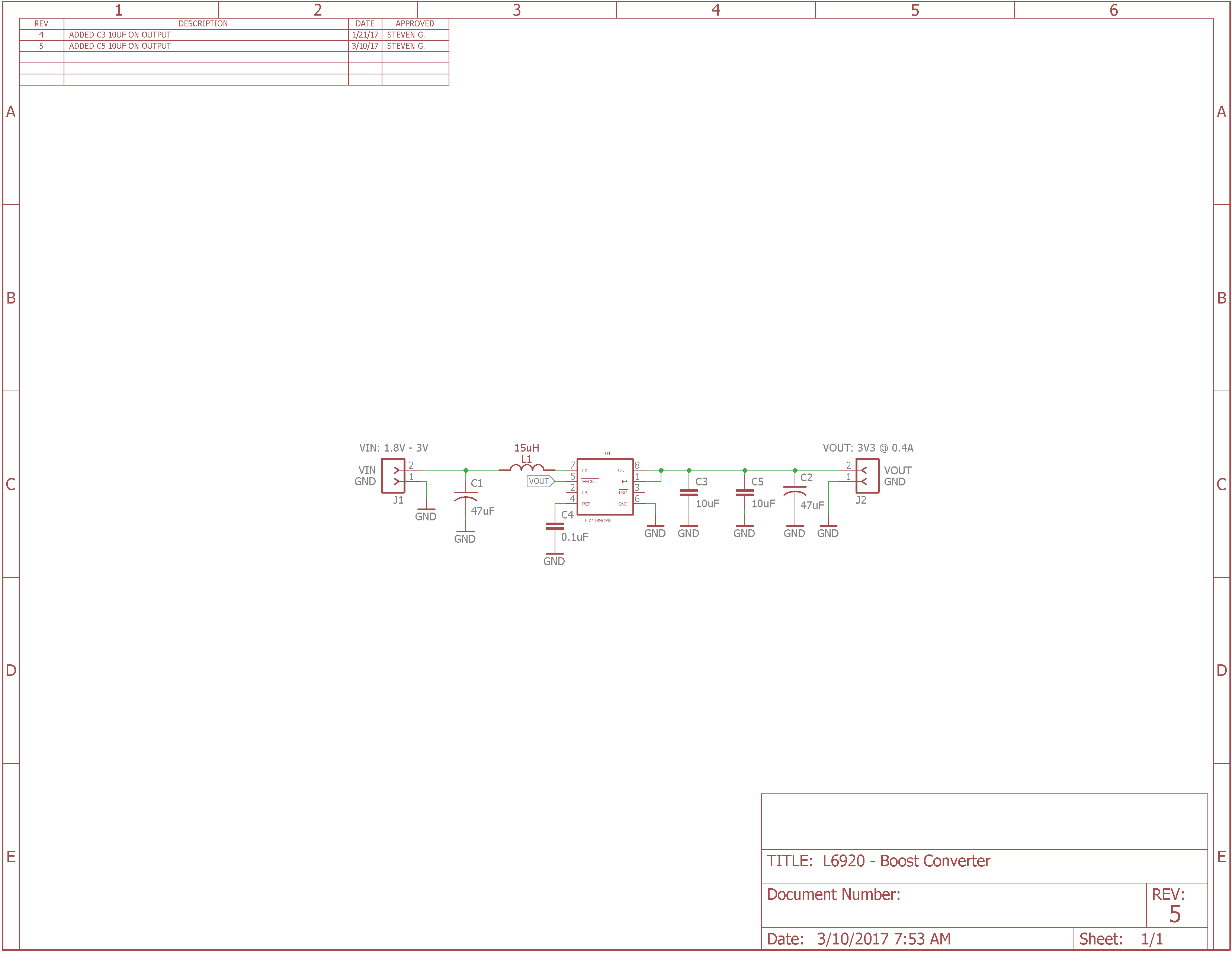Layout.

I figure I’d help you guys out a bit if I added the layout for this board.  My approach for this layout was to minimize the overall size in order to get a better price for manufacturing the board.Testing.

Here comes the fun part, actually testing what you designed.  Now one thing that took me awhile to learn was that design and theory never really match reality.  There are a lot of different parameters that are not accounted for when designing in theory.

A couple of the major issues that could make or break your design is parasitic elements.  One of the biggest parasitic elements is ESR for output capacitors.  This is the equivalent series resistance of the capacitor that is not taking into account when designing.  In my post that covers the design of a boost converter, I emphasizes this topic to make you aware of this parasitic element.

Now, my design parameters consisted of loading the converter at 3 different voltage inputs (1.8V, 2.0V, and 2.4V).  Each input voltage was loaded starting at 0.1A and ending at 0.4A.  This load all depended on which input voltage was tested because the lower input voltage cannot provide the max output power.

First test – Vin: 1.8V @ 0.2A

Next test – Vin: 2.0V @ 0.3A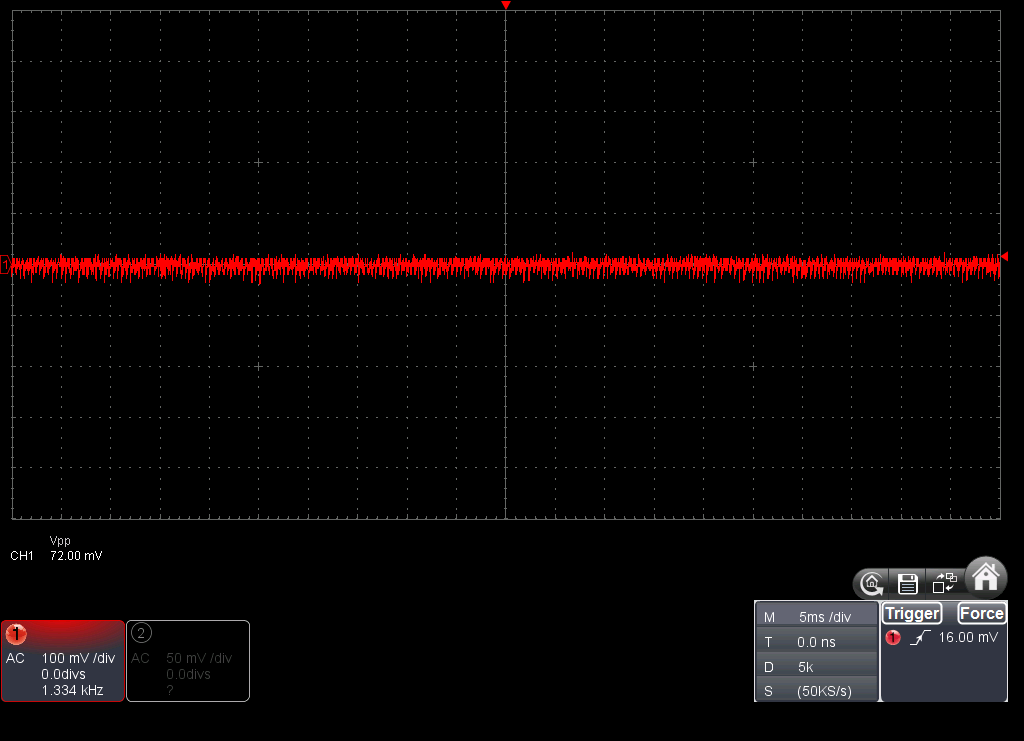Last test – Vin: 2.4V @ 0.4A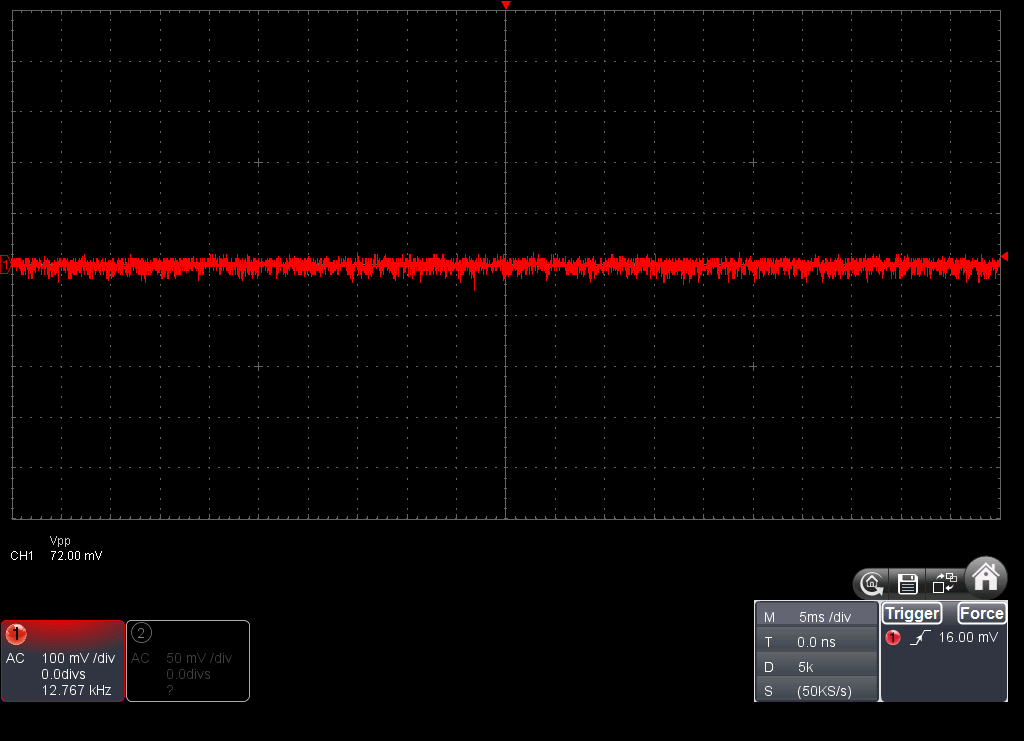After completely the voltage ripple test, I also conducted a load regulation test at max load for each input voltage.  I got a 1.5% voltage drop from calculated voltage meaning at full load, my output voltage was 3.25V at the lowest.

All files available here – Click

## How-to: Design a Boost Converter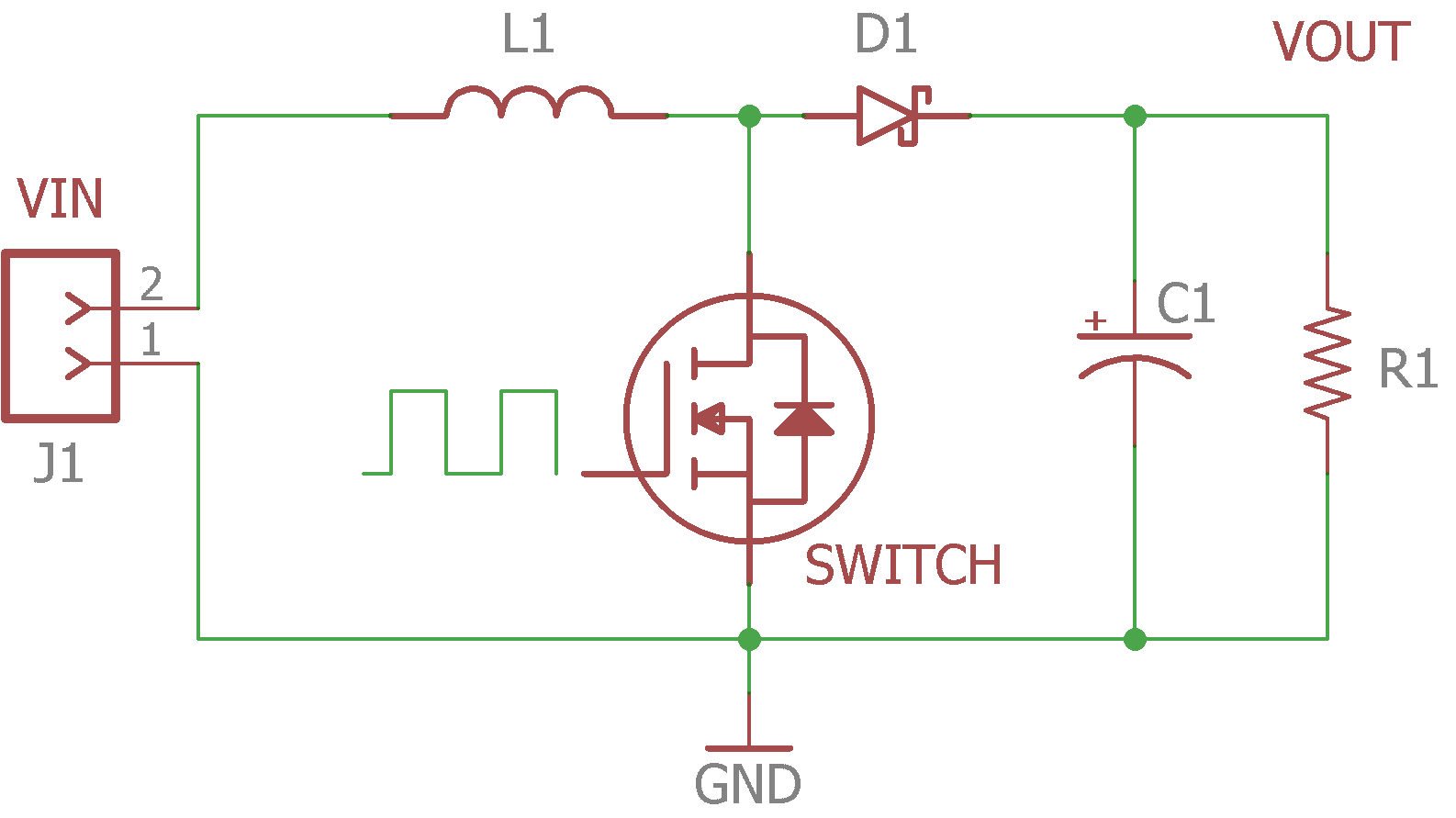Figure 1: Basic Boost Converter Circuit

#### Designing a boost converter sounds complicated and intimidating, well that was always my impression when it came to this topic in school.  In reality, the design and testing of a boost converter is a lot easier than meets the eye.

Here I will walk you step by step on designing your first boost converter and how the datasheet is your best friend when designing.  For this tutorial we will be using the L6920DC IC Boost converter from skyworks.

This information was referenced from TI reference report.

First and foremost, download the highlighted datasheet, datasheet-l6920dc. This has all the highlighted paremeters that you will need when designing a boost converter.

Step 1:

You need to decide what are your specifications.  These are the key parameters:

• Vin(min)
• Vin(max)
• Vout
• Iout
• n = efficiency; Most boost converters average around 85 to 90% under medium load and up to 95% on heavy load.  We will use the lowest percentage to be safe.

Example:

• Vin(min): 1.8V
• Vin(max): 2.4V
• Vout: 3.3V
• Iout: 0.4A
• n = 87% or 0.87

Step 2:

With your specifications, next step is to find your DUTY CYCLE:$\bf \Huge D= 1 - \cfrac{(V_{\text{IN}}*n)}{V_{\text{OUT}}}$

We calculated the duty cycle for both lowest input voltage and highest input voltage.

• Lowest input voltage gives you the highest switching current you will see
• Highest input voltage gives you the highest output current your converter can produce

Example:$\bf \Huge V_{\text{IN-MIN}}$$\Huge D = 1 - \cfrac{1.8V*0.85}{3.3V}$ = 0.52$\bf \Huge V_{\text{IN-MAX}}$$\Huge D = 1 - \cfrac{2.4V*0.85}{3.3V}$ = 0.36

Step 3:

Next we will estimate the switching current or CURRENT RIPPLE of the Inductor:

ΔIL =$(0.3) * I_\text{OUTmax} * \cfrac{(V_\text{OUT})}{(V_\text{IN})}$

Example:$\bf \Huge V_{\text{IN-MIN}}$

ΔIL =$\Huge (0.3) * 0.4A * \cfrac{3.3V}{1.8V}$ = 0.22A$\bf \Huge V_{\text{IN-MAX}}$

ΔIL =$\Huge (0.3) * 0.4A * \cfrac{3.3V}{2.4V}$ = 0.165A

Step 4:

Next we calculate the minimum INDUCTANCE we need:$\bf \Huge L_\text{MIN} = \cfrac{(V_\text{IN})*(V_\text{OUT} - V_\text{IN})}{\Delta I_\text{L}*f_\text{S}*V_\text{OUT}}$$\bf \Huge f_\text{S}$ – This is the switching frequency that the converter will operate at.

Example:$\bf \Huge V_{\text{IN-MIN}}$$\Huge L_\text{MIN} = \cfrac{(1.8V)*(3.3V - 1.8V)}{0.22A*1MHz*3.3V}$ = 3.72uH$\bf \Huge V_{\text{IN-MAX}}$$\Huge L_\text{MIN} = \cfrac{(2.4V)*(3.3V - 2.4V)}{0.165A*1MHz*3.3V}$ = 3.97uH

We would select the highest inductance value to meet our input voltage rage of 1.8V-2.4V

When selecting the inductor, the key parameters you need to look for is low DCR, package size, and max current the inductor can handle.

DCR – Is the resistance in the coil because at the end of the day, an inductor is still a wire. When you keep this value at a minimum, it will increase your effieciency and the ability to provide a higher output power.

In step 7 , you will calculate the maximum current the inductor will see and there you will have all the necessary parameters needed to chose the inductor.

Step 5:

Now that we have our inductor value, we can calculate the actual CURRENT RIPPLE of the Inductor:

ΔIL =$\bf \Huge \cfrac{V_{IN}*D}{f_\text{S}*L}$

Example:$\bf \Huge V_{\text{IN-MIN}}$

ΔIL =$\Huge \cfrac{1.8V*0.525}{1MHz*3.72uH}$ = 0.19A$\bf \Huge V_{\text{IN-MAX}}$

ΔIL =$\Huge \cfrac{2.4V*0.36}{1MHz*3.97uH}$ = 0.18A

Step 6:

Next we need to calculate the MAX OUTPUT CURRENT the boost converter can output:$\bf \Huge I_\text{MAXOUT}$ =$\bf \Huge (I_\text{LIM} - \cfrac{\Delta I_\text{L}}{2}) * (1 - D)$$I_\text{LIM}$ – This is the current switch limit of the boost converter.

Example:$\bf \Huge V_{\text{IN-MIN}}$$\Huge I_\text{MAXOUT}$ =$\bf \Huge (0.8A - \cfrac{0.19A}{2}) * (1 - 0.52)$ = 0.33A$\bf \Huge V_{\text{IN-MAX}}$$\Huge I_\text{MAXOUT}$ =$\bf \Huge (0.8A - \cfrac{0.18A}{2}) * (1 - 0.36)$ = 0.45A

Step 7:

Next we will calculate the MAX SWITCHING CURRENT,$I_\text{SW}$ the Inductor will see.  This value cannot exceed the ILIM value of the boost converter:$\bf \Huge I_\text{SW-MAX} = \cfrac{\Delta I_\text{L}}{2} + \cfrac{I_\text{OUT}}{1 - D}$

Example:$\bf \Huge V_{\text{IN-MIN}}$$\Huge I_\text{SW-MAX} = \cfrac{0.19A}{2} + \cfrac{0.4A}{1 - 0.525}$ = 0.94A$\bf \Huge V_{\text{IN-MAX}}$$\Huge I_\text{SW-MIN} = \cfrac{0.18A}{2} + \cfrac{0.4A}{1 - 0.36}$ = 0.72A

Note:$\Huge I_\text{SW-MAX}$ value cannot exceed$\Huge I_\text{LIM}$ which can be found in the datasheet.  In this example we see that with a low input voltage, the switching current exceeds the limit in the datasheet.  The boost converter might still be able to output the desired current at that low input voltage because$\Huge I_\text{LIM}$ is the minimum switching current it can handle.  But better to be safe than sorry.

Here you can see the inductor will see a max of 0.94A at its lowest input voltage. Now we can chose the inductor for our design.

For this design I went with,MSS5131-472MLB, a 4.7uH inductor from coilcraft.

Since I chose an inductor that has a higher value than previous calculated, the inductor current ripple and output power will be slightly lower but it will not effect your design negatively.

Step 8:

This step is only if your boost converter has an adjustable output voltage.

(This boost converter is a fixed output and does not require these resistors.  Step 8 values are dummy values but the process )

Here we will find R1 AND R2 values for the feedback network:$\bf \Huge I_\text{R0.5} >= 100 * I_\text{FB}$$\bf \Huge I_\text{FB}$ – This is the current that the feedback resistor draws.$\bf \Huge R_2 = \cfrac{V_\text{FB}}{I_\text{R0.5}}$$\bf \Huge V_\text{FB}$ – This is the feedback reference voltage$\bf \Huge R_1 = R_2 * (\cfrac{V_\text{OUT}}{V_\text{FB}}-1)$

Example:$\Huge I_\text{R0.5} >= 100 * 350nA$ = 35mA$\Huge R_2 = \cfrac{1.24V}{35mA}$ = 35.4kΩ$\Huge R_1 = 35.4k \Omega * (\cfrac{3.3V}{1.24V}-1)$ = 58.74kΩ

Step 9:

Next l, we will calculate the INPUT CAPACITOR and OUTPUT CAPACITOR needed to minimize the ripple going in and out of the system:

First, you find your input capacitor:$\bf \Huge C_\text{IN}$: Typically this value is 4.7uF to 10uF

Next, we need to first to look at these two equations below:$\bf \Huge \Delta V_\text{OUT}= \cfrac{I_\text{OUT} *T_\text{ONmax}}{ C_\text{OUT}}$$\bf \Huge T_\text{ONmax}$ – This is the maximum on time of the boost converter.  It is also written as$\bf \Huge D * T_\text{S}$

Were$\bf \Huge T_\text{S} = \cfrac{1}{f_\text{S}}$$\bf \Huge \Delta V_\text{OUTesr} = ESR* I_\text{SW-MAX}$

ESR – All capacitors are not ideal capacitors and therefore have what is known as Equivalent Series Resistance. This is an important parameter that you need to consider when choosing the right output capacitor.

Example:

Cin = 10uF

First, we need to choose a voltage ripple that we can live with. Here I chose 50mV, and if we rearrange the first equation, we get:$\Huge \Delta C_\text{OUTmin}= \cfrac{0.4 *6.25us}{50mV}$ = 50uF

Now we have a couple of options to choose from when it comes to materials for capacitors.

Most common are ceramic and electrolytic capacitors.  Each have there own pro and con.

Ceramic capacitors offer lower ESR for lower ripple but they typically do not have the bulk capacitance.

Electrolytic capacitors have bulk capacitance but generally have a high ESR that adds to ripple.

In this case I decided to go with both, getting the benefit of bulk capacitance and low ESR.

I went with a 1206 package, 10uF ceramic capacitor and a 47uF Electrolytic in parallel. For the electrolytic, they also have an aluminum polymer that has high capacitance with the added benefit of low ESR. I went with a 47uF that has an ESR of 40mΩ.

Now we plug in the values we got back into the equations and we get:Special Note: For ceramic capacitors, you need to be careful of which class and package size you choose because you only see a certain percentage of your nominal value (ex. 1206 10uF X7R will see 73% of 10uF). Click here for more info. I generally go with 1206 or 1210 with capacitors.$\Huge \Delta V_\text{OUT}= \cfrac{0.4A * 6.25us}{50uF}$ = 50mV$\Huge \Delta V_\text{OUTesr} = 40m\Omega*0.94A$ = 37mV$\Huge \Delta V_\text{OUT}$ = 87mV

Always refer to the datasheet and compare recommended value vs calculated

You’ve now designed your own boost converter regulator.  See it wasnt too hard :).

I will post this project soon that has the schematic and bill of materials, it’ll be under the projects menu bar, stay tuned!!

Feel free to comment below and correct me if anything seems incorrect to you.

References:

5. Ceramic or electrolytic output capacitors